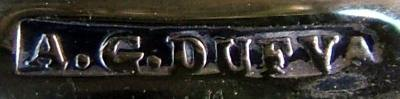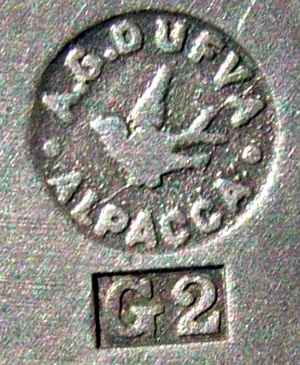ASSOCIATION OF SMALL COLLECTORS OF ANTIQUE SILVER
ASCAS
 Members' Window # 84/1 home SITE MAP
by Prof. David N. Nikogosyan, University College Cork, Cork, Ireland

## MARKS OF EUROPEAN SILVER PLATE: VIII. DUFVA, SWEDEN

##### I think that the most interesting information for the ASCAS collectors/readers is the system of the production year marking, invented by A.G. Dufva. He took 24 letters from the Latin alphabet and referred them to 24 years, starting from 1882, in the following sequence: A = 1888, B = 1883, C = 1884, D = 1885, E = 1886, F = 1887, G = 1888, H = 1889, I = 1890, K = 1891, L = 1892, M = 1893, N = 1894, O = 1895, P = 1896, Q = 1897, R = 1898, S = 1899, T = 1900, U = 1901, V = 1902, X = 1903, Y = 1904, Z = 1905 This sequence of letters was repeated after 1905 with a digit 2 added to each letter, that means: A2 = 1906, B2 = 1907, C2 = 1908, D2 = 1909, E2 = 1910, F2 = 1911, G2 = 1912, H2 = 1913, I2 = 1914, K2 = 1915, L2 = 1916, M2 = 1917, N2 = 1918, O2 = 1919, P2 = 1920, Q2 = 1921, R2 = 1922, S2 = 1923, T2 = 1924, U2 = 1925, V2 = 1926, X2 = 1927, The production year marking after 1927 is not known. Some typical Dufva marks for silver plate (since 1897 the the company also produced sterling items) are shown below. I am grateful to Mr. Anders Wigh, who gave me the first information about the Dufva foundry. I also want to thank Ms. Helena Sergeyev for her translation from Swedish and Dr. Lars Lindqvist for his involvement. The author is also indebted to Mr. Chris Turner who sent him a photograph of Dufva item issued in 1927.

Some Dufva marks
MARK

COMMENT

1##### Marked by three inscriptions: 1) "A.G.DUFVA", which means Axel Gabriel Dufva, the length of "DUFVA" word is 4.6 mm, the ratio of the word’s length to its height is 5.5; 2) "NS", which means nickel silver and 3) "G", which means 1888. This mark was in use at least between 1888 and 1896.

2